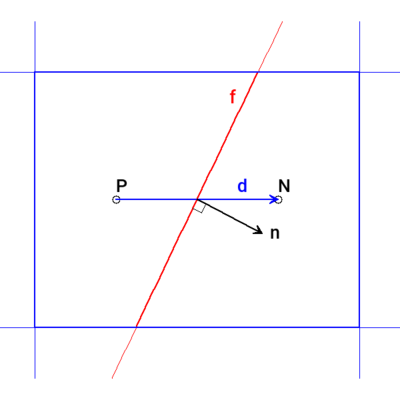The full gradient of a property $$Q$$ at a face can can be interpolated from the cell-based gradient. The surface-normal contribution is then represented by:

$\snGrad Q = \vec{n} \dprod \left( \grad Q \right)_f$

where $$\vec{n}$$ is the face unit normal. The stencil for the gradient calculation at the face, $$f$$, between cells P and N is described by the following figure:where the vector $$\vec{d}$$ joins the two cell centres. A variety of schemes are available that differ in their application based on the angle, $$\theta$$, between the $$\vec{d}$$ and $$\vec{n}$$ vectors, representing the degree of non-orthogonality.

## Usage🔗

Surface-normal gradient schemes are specified in the fvSchemesfile under the snGradSchemes sub-dictionary using the syntax:

snGradSchemes
{
default         none;